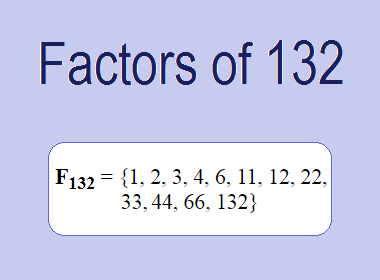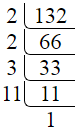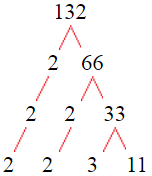# Factors of 132The factors of 132 are 1, 2, 3, 4, 6, 11, 12, 22, 33, 44, 66, and 132 i.e. F132 = {1, 2, 3, 4, 6, 11, 12, 22, 33, 44, 66, 132}. The factors of 132 are all the numbers that can divide 132 without leaving a remainder.

We can check if these numbers are factors of 132 by dividing 132 by each of them. If the result is a whole number, then the number is a factor of 132. Let's do this for each of the numbers listed above:

·        1 is a factor of 132 because 132 divided by 1 is 132.

·        2 is a factor of 132 because 132 divided by 2 is 66.

·        3 is a factor of 132 because 132 divided by 3 is 44.

·        4 is a factor of 132 because 132 divided by 4 is 33.

·        6 is a factor of 132 because 132 divided by 6 is 22.

·        11 is a factor of 132 because 132 divided by 11 is 12.

·        12 is a factor of 132 because 132 divided by 12 is 11.

·        22 is a factor of 132 because 132 divided by 22 is 6.

·        33 is a factor of 132 because 132 divided by 33 is 4.

·        44 is a factor of 132 because 132 divided by 44 is 3.

·        66 is a factor of 132 because 132 divided by 66 is 2.

·        132 is a factor of 132 because 132 divided by 132 is 1.

## How to Find Factors of 132?

1 and the number itself are the factors of every number. So, 1 and 132 are two factors of 132. To find the other factors of 132, we can start by dividing 132 by the numbers between 1 and 132. If we divide 132 by 2, we get a remainder of 0. Therefore, 2 is a factor of 132. If we divide 132 by 3, we get a remainder of 0. Therefore, 3 is also a factor of 132.

Next, we can check if 4 is a factor of 132. If we divide 132 by 4, we get a remainder of 0. Therefore, 4 is a factor of 132. We can continue this process for all the possible factors of 132.

Through this process, we can find that the factors of 132 are 1, 2, 3, 4, 6, 11, 12, 22, 33, 44, 66, and 132. These are the only numbers that can divide 132 without leaving a remainder.

********************

********************

## Properties of the Factors of 132

The factors of 132 have some interesting properties. One of the properties is that the sum of the factors of 132 is equal to 336. We can see this by adding all the factors of 132 together:

1 + 2 + 3 + 4 + 6 + 11 + 12 + 22 + 33 + 44 + 66 + 132 = 336

Another property of the factors of 132 is that the prime factors of 132 are 2, 3 and 11 only.

## Applications of the Factors of 132

The factors of 132 have several applications in mathematics. One of the applications is in finding the highest common factor (HCF) of two or more numbers. The HCF is the largest factor that two or more numbers have in common. For example, to find the HCF of 132 and 55, we need to find the factors of both numbers and identify the largest factor they have in common. The factors of 132 are 1, 2, 3, 4, 6, 11, 12, 22, 33, 44, 66, and 132. The factors of 55 are 1, 5, 11, and 55. The largest factor that they have in common is 11. Therefore, the HCF of 132 and 55 is 11.

Another application of the factors of 132 is in prime factorization. Prime factorization is the process of expressing a number as the product of its prime factors. The prime factors of 132 are 2, 3 and 11, since these are the only prime numbers that can divide 132 without leaving a remainder. Therefore, we can express 132 as:

132 = 2 × 2 × 3 × 11

We can do prime factorization by division and factor tree method also. Here is the prime factorization of 132 by division method,132 = 2 × 2 × 3 × 11

Here is the prime factorization of 132 by the factor tree method,132 = 2 × 2 × 3 × 11

## Conclusion

The factors of 132 are the numbers that can divide 132 without leaving a remainder. The factors of 132 are 1, 2, 3, 4, 6, 11, 12, 22, 33, 44, 66, and 132. The factors of 132 have some interesting properties, such as having a sum of 336. The factors of 132 have several applications in mathematics, such as finding the highest common factor and prime factorization.